JEE  >  Test: MCQs (One or More Correct Option): Circle | JEE Advanced

# Test: MCQs (One or More Correct Option): Circle | JEE Advanced

Test Description

## 6 Questions MCQ Test Maths 35 Years JEE Main & Advanced Past year Papers | Test: MCQs (One or More Correct Option): Circle | JEE Advanced

Test: MCQs (One or More Correct Option): Circle | JEE Advanced for JEE 2023 is part of Maths 35 Years JEE Main & Advanced Past year Papers preparation. The Test: MCQs (One or More Correct Option): Circle | JEE Advanced questions and answers have been prepared according to the JEE exam syllabus.The Test: MCQs (One or More Correct Option): Circle | JEE Advanced MCQs are made for JEE 2023 Exam. Find important definitions, questions, notes, meanings, examples, exercises, MCQs and online tests for Test: MCQs (One or More Correct Option): Circle | JEE Advanced below.
 1 Crore+ students have signed up on EduRev. Have you?
*Multiple options can be correct
Test: MCQs (One or More Correct Option): Circle | JEE Advanced - Question 1

### The equations of the tangents drawn from the origin to the circle x2 + y2 – 2rx – 2hy + h2 = 0, are (1988 - 2 Marks)

Detailed Solution for Test: MCQs (One or More Correct Option): Circle | JEE Advanced - Question 1

The given circle is x2 + y2 – 2rx – 2hy + h2 = 0 with centre (r, h) and radius = r.
Clearly circle touches y-axis so one of its tangent is x = 0.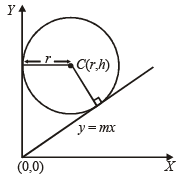Let y =  mx be the other tangent through origin.
Then length of perpendicular from C (r, h) to y = mx should be equal to r.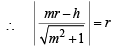⇒ m2r2 – 2mrh + h2 = m2r2 + r2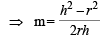∴ Other tangent is y =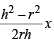or (h2 – r2) x – 2rhy = 0

*Multiple options can be correct
Test: MCQs (One or More Correct Option): Circle | JEE Advanced - Question 2

### The number of common tangents to the circles x2 + y2  = 4 and x2 + y2 – 6x – 8y = 24 is (1998 - 2 Marks)

Detailed Solution for Test: MCQs (One or More Correct Option): Circle | JEE Advanced - Question 2

x2 + y2 = 4 (given) Centre C1 ≡ (0, 0) and R1 = 2.
Also for circle x2 + y2 – 6x – 8y – 24 = 0 C2 ≡ (3, 4) and R2 = 7.
Again C1 C2 = 5 = R2 – R1

Therefore, the given circles touch internally such that they can have just one common tangent at the point of contact.

*Multiple options can be correct
Test: MCQs (One or More Correct Option): Circle | JEE Advanced - Question 3

### If the circle x2 + y2 = a2 intersects the hyperbola xy = c2 in four points P(x1, y1), Q(x2, y2), R(x3, y3), S(x4, y4), then (1998 - 2 Marks)

Detailed Solution for Test: MCQs (One or More Correct Option): Circle | JEE Advanced - Question 3

Putting y = c2/x in x2 + y2 = a2,
we obtain x2 + c4/x2 = a2

⇒ x4 – a2x2 + c4 = 0 … (1)
As x1, x2, x3 and x4 are roots of (1),
⇒ x1 + x2 + x3 + x4 = 0 and x1 x2 x3 x4 = c4
Similarly, forming equation in y,
we get y1 + y2 + y3 + y4 = 0 and y1 y2 y3 y4 = c4.

*Multiple options can be correct
Test: MCQs (One or More Correct Option): Circle | JEE Advanced - Question 4

Circle(s) touching x-axis at a distance 3 from the origin and having an intercept of length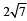on y-axis is (are) (JEE Adv. 2013)

Detailed Solution for Test: MCQs (One or More Correct Option): Circle | JEE Advanced - Question 4

There can be two possibilites for the given circle as shown in the figure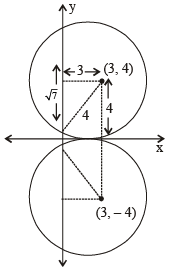∴  The equations of circles can be (x – 3)2 + (y – 4)2 = 42
or  (x – 3)2 + (y + 4)2 = 42
i.e.  x2 + y2 – 6x – 8y + 9 = 0
or   x2 + y2 – 6x + 8y + 9 = 0

*Multiple options can be correct
Test: MCQs (One or More Correct Option): Circle | JEE Advanced - Question 5

A circle S passes through the point (0, 1) and is orthogonal to the circles (x – 1)2 + y2 = 16 and x2 + y2 = 1. Then (JEE Adv. 2014)

Detailed Solution for Test: MCQs (One or More Correct Option): Circle | JEE Advanced - Question 5

Let the equation of circle be x2 + y2 + 2gx + 2 f y + c = 0
It passes through (0, 1)
∴ 1 + 2f + c = 0 ...(i)
This circle is orthogonal to (x – 1)2 + y2 = 16
i.e. x2 + y2 – 2x – 15 = 0 and x2 + y2 – 1 = 0
∴ We should have 2g (– 1) + 2f (0) = c – 15 or 2g + c – 15 = 0 ...(ii)
and 2g(0) + 2f (0) = c – 1 or c = 1 ...(iii)
Solving (i), (ii) and (iii), we get c = 1, g  = 7, f = – 1
∴ Required circle is x2 + y2 + 14x – 2y + 1 = 0
With centre (– 7, 1) and radius = 7
∴ (b) and (c) are correct options.

*Multiple options can be correct
Test: MCQs (One or More Correct Option): Circle | JEE Advanced - Question 6

Let RS be the diameter of the circle x2 + y2 = 1, where S is the point (1, 0). Let P be a variable point (other than R and S) on the circle and tangents to the circle at S and P meet at the point Q. The normal to the circle at P intersects a line drawn through Q parallel to RS at point E. Then the locus of E passes through the point(s) (JEE Adv. 2016)

Detailed Solution for Test: MCQs (One or More Correct Option): Circle | JEE Advanced - Question 6

Circle : x2 + y2 = 1
Equation of tangent at P(cosθ, sinθ) x cosθ + y sinθ = 1 ...(1)
Equation of normal at P y = x tanθ ...(2)
Equation of tangent at S is x = 1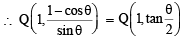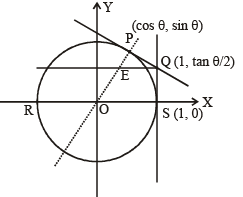∴ Equation of line through Q and parallel to RS is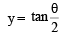∴ Intersection point E of normal and= x tanθ ⇒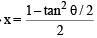∴ Locus of E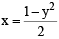or y2 = 1 – 2x

It is satisfied by the points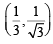and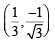## Maths 35 Years JEE Main & Advanced Past year Papers

132 docs|70 tests
 Use Code STAYHOME200 and get INR 200 additional OFF Use Coupon Code
Information about Test: MCQs (One or More Correct Option): Circle | JEE Advanced Page
In this test you can find the Exam questions for Test: MCQs (One or More Correct Option): Circle | JEE Advanced solved & explained in the simplest way possible. Besides giving Questions and answers for Test: MCQs (One or More Correct Option): Circle | JEE Advanced, EduRev gives you an ample number of Online tests for practice

## Maths 35 Years JEE Main & Advanced Past year Papers

132 docs|70 tests